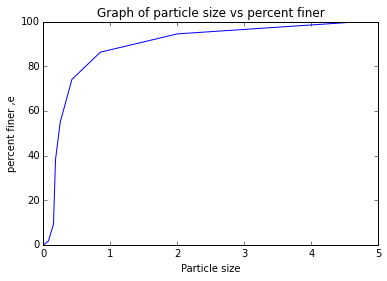# Chapter2-Origin of Soil and Grain Size¶

## Ex1-pg44¶

In :
#calculate the values and plot the graph
%matplotlib inline
import warnings
warnings.filterwarnings('ignore')
import math

from math import log
import numpy
from math import tan
import matplotlib
from matplotlib import pyplot
#given
e=numpy.array([100,94.5,86.3,74.1,54.9,38.1,9.3,1.7,0])
p=numpy.array([4.75,2.00,0.850,0.425,0.250,0.180,0.150,0.075,0])

#calculations

#results

pyplot.plot(p,e)
pyplot.xlabel('Particle size ')
pyplot.ylabel('percent finer ,e')
pyplot.title('Graph of particle size vs percent finer')
pyplot.show()
print('look at the axis reverse in text book')look at the axis reverse in text book


## Ex2-pg45¶

In :
#calculate uniformity coefficient and coefficient of gradation
##initialisation of variables
##from graph
d= 0.15 ##mm
w= 0.17 ##mm
a= 0.27 ##mm
##calculations
C= a/d
c= w**2/(a*d)
##results
print'%s %.1f %s'%('uniformity coefficient = ',C,"")
print'%s %.2f %s'% ('coefficient of gradation = ',c,' ')

uniformity coefficient =  1.8